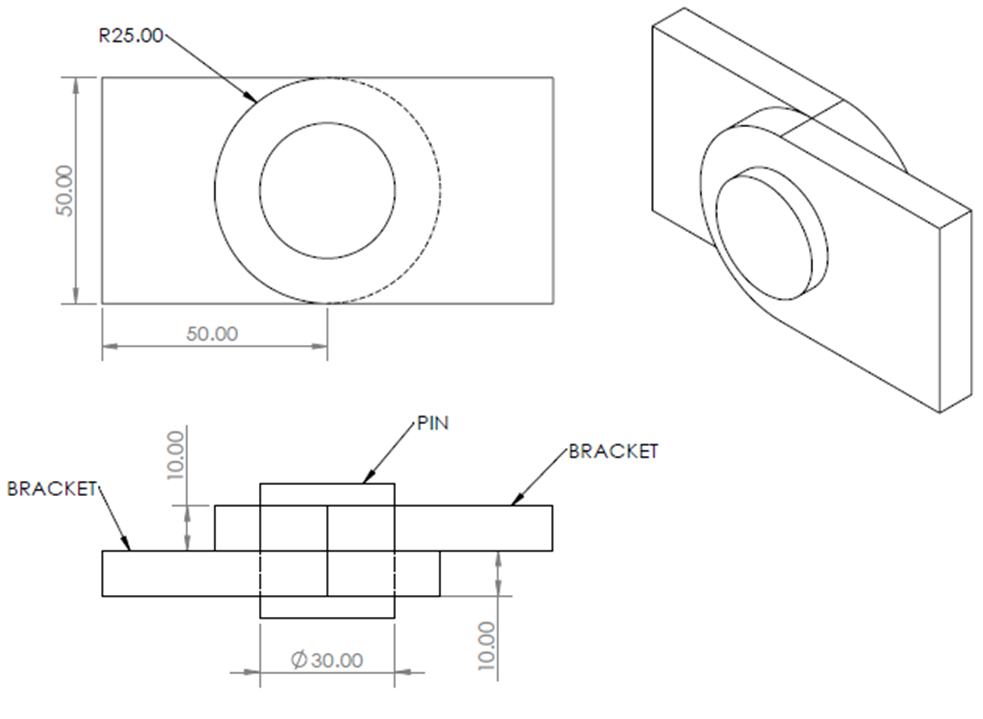## Joint Shear Stress

Consider the below joint is made up of two titanium brackets that are pinned together in single shear with a 30 mm pin. The brackets are 50 N in tension, which causes the pin to experience a 10 kN tension. What is the average shear stress at the overlapping brackets’ interface? Unless otherwise specified, all units are in mm.Hint
Shear stress:
$$\tau=\frac{T}{A}$$$where $$T$$ is the shearing force, and $$A$$ is the area. Hint 2 $$A=\pi (r_{outer}-r_{inner})^2$$$
where $$A$$ is the area and $$r$$ is the radius.
Shear stress:
$$\tau=\frac{T}{A}$$$where $$T$$ is the shearing force, and $$A$$ is the area. To find $$A$$ : $$A=\pi (r_{outer}-r_{inner})^2$$$
where $$A$$ is the area and $$r$$ is the radius. Thus,
$$A=\pi[(25mm)^2-(\frac{30mm}{2})^2]=\pi[625mm^2-225mm^2]=1,256\:mm^2$$$Finally, $$\tau=\frac{50\:N}{1,256\:mm^2}=0.04\:MPa$$$
0.04 MPa